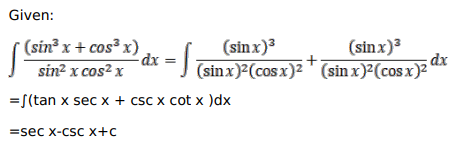# Mark against the correct answer in each of the following:

Question:

Mark $(\sqrt{)}$ against the correct answer in each of the following:

$\int \frac{\left(\sin ^{3} x+\cos ^{3} x\right)}{\sin ^{2} x \cos ^{2} x} d x=?$

A. $\sin x-\cos x+C$

B. $\tan x-\cos x+C$

C. $\sec x-\operatorname{cosec} x+C$

D. None of these

Solution: